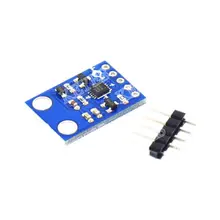MSP-EXP432P401R LaunchPad and MMA7660FC accelerometer example

In this example we show how to connect a MMA7660FC accelerometer to a MSP-EXP432P401R LaunchPad, the example will use the Energia IDE.

I used a MMA7660FC module in this example, which you can see belowThe MMA7660FC is a digital output I²C, very low-power, low-profile capacitive micro-machined accelerometer featuring a low pass filter, compensation for zero-g offset and gain errors and conversion to six-bit digital values at a user configurable output data rate. The device can be used for sensor data changes, product orientation and gesture detection through an interrupt pin (INT).

Connection

Here is a picture of the launchpad so you can see what pins we are referring to below in the table

 Module Connection MSP432 Connection SDA J1-10 SDA SCL J1-9 SCL Gnd J3-22 Gnd Vcc J1-1 3.3v

Code

 Source code```#include <Wire.h>

// MMA7660FC I2C address is 0x4C(76)

void setup()
{
// Initialise I2C communication as MASTER
Wire.begin();
// Initialise Serial Communication, set baud rate = 9600
Serial.begin(9600);

// Start I2C Transmission
// Select mode register
Wire.write(0x07);
// Select active mode
Wire.write(0x01);
// Stop I2C Transmission
Wire.endTransmission();

// Start I2C Transmission
// Select sample rate register register
Wire.write(0x08);
// 1 sample per second
Wire.write(0x07);
// Stop I2C Transmission
Wire.endTransmission();
delay(300);
}

void loop()
{
unsigned int data;

// Start I2C Transmission
// Select Data Register
Wire.write(0x00);
// Stop I2C Transmission
Wire.endTransmission();

// Request 3 bytes of data

// xAccl, yAccl, zAccl
if(Wire.available() == 3)
{
}

// Convert the data to 6-bits
int xAccl = data & 0x3F;
if(xAccl > 31)
{
xAccl -= 64;
}
int yAccl = data & 0x3F;
if(yAccl > 31)
{
yAccl -= 64;
}
int zAccl = data & 0x3F;
if(zAccl > 31)
{
zAccl -= 64;
}

// Output data to serial monitor
Serial.print("Acceleration in X-Axis :");
Serial.println(xAccl);
Serial.print("Acceleration in Y-Axis :");
Serial.println(yAccl);
Serial.print("Acceleration in Z-Axis :");
Serial.println(zAccl) ;
delay(500);
}```

Output

Open the serial monitor and you should see something like this

Acceleration in X-Axis :-10
Acceleration in Y-Axis :-6
Acceleration in Z-Axis :17
Acceleration in X-Axis :-10
Acceleration in Y-Axis :-6
Acceleration in Z-Axis :18
Acceleration in X-Axis :-11
Acceleration in Y-Axis :-6
Acceleration in Z-Axis :17
Acceleration in X-Axis :-12
Acceleration in Y-Axis :-7
Acceleration in Z-Axis :18